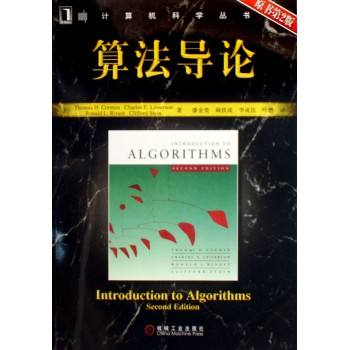# 求职 | 华为研发工程师编程题个人题解【C++】【Python】——————算法—————— 同时被 2 个专栏收录13 篇文章 0 订阅

### 问题1

输入文件最多包含10组测试数据，每个数据占一行，仅包含一个正整数n（1<=n<=100），表示小张手上的空汽水瓶数。n=0表示输入结束，你的程序不应当处理这一行。


对于每组测试数据，输出一行，表示最多可以喝的汽水瓶数。如果一瓶也喝不到，输出0。


输入：
10

5


### 代码

C++版

#include<cstdio>
using namespace std;

int main(){
int n;
while(scanf("%d",&n) != EOF){
if(n == 0){
return 0;
}
if(n == 1){
printf("1\n");
}
else if(n == 2){
printf("3\n");
}
else{
int cnt = 0;
while(n/3 != 0){
int temp = n/3;
cnt += n/3;
n %= 3;
n += temp;
}
if(n == 2){
cnt += 1;
printf("%d\n", cnt);
}
else{
printf("%d\n", cnt);
}
}
}
return 0;
}


Python版

def drink():
while True:
try:
num = int(input())
if num != 0:
print(num//2)  # 整除2
except:
break

if __name__ == "__main__":
drink()


### 问题2

Input Param

n        输入随机数的个数

inputArray   n个随机整数组成的数组

Return Value

OutputArray  输出处理后的随机整数


样例输入解释：



输入多行，先输入随机整数的个数，再输入相应个数的整数


返回多行，处理后的结果


输入：
3
2
2
1

1
2
2


### 代码

C++版

#include<cstdio>
#include<algorithm>
using namespace std;

int main(){
int n;
int num;
while(scanf("%d", &n) != EOF){
bool flag = {false};  //每次循环都要更新
for(int i = 0; i < n; ++i){
scanf("%d", &num);
flag[num] = true;
}
for(int i = 0; i < 1001; ++i){
if(flag[i] == true){
printf("%d\n", i);
}
}
}
return 0;
}


Python版

def number():
while True:
try:
num, res = int(input()), set()
for i in range(num):
for i in sorted(res):
print(i)
except:
break

if __name__ == "__main__":
number()


### 问题3

输入一个十六进制的数值字符串。


输出该数值的十进制字符串。


输入：
0xA

10


### 思路

C++ 和 Python 都有自带的进制转换函数，详情可以直接看 C++版 法一 和 Python版 代码。

### 代码

C++版

#include<iostream>
#include<cstdio>
#include<string>
#include<cmath>
using namespace std;

int main(){
/*
//法一
int num;
while((cin >> hex >> num)){
cout << num << endl;
}
*/

//法二
string s;
while((cin >> s)){
int len = s.length();
int num = 0;
for(int i = len-1; i >= 0; --i){
if(s[i]>='0' && s[i]<='9'){  //数字字符的转换
num+=(s[i]-48)*pow(16,len-i-1);
}
else if(s[i]>='A' && s[i]<='F'){  //字母字符的转换
num+=(s[i]-55)*pow(16,len-i-1);
}
}
printf("%d\n", num);
}
return 0;
}


Python版

import sys

def sixteen():
while True:
try:
print(int(string_hex, 16))
except:
break

if __name__ == "__main__":
sixteen()

11-271万+09-051万+
03-289548
07-242万+
09-164732
09-196611
01-18109
06-1789
10-1590
10-06914
06-2799
04-26
09-221580
12-091万+
09-092万+
03-06
06-224995点击重新获取扫码支付余额充值